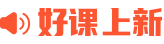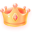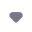¥20.00 26 节 · 125 人已学阳兴旺matlab导数计算
matlab导数计算，通过编程实战掌握具体应用。包括matlab导数计算前、matlab导数计算中、matlab导数计算后。
¥12.00超级会员免费
3 节 · 93 人已学硬核野生技术咨询客服小李matlab极限
matlab极限，通过编程实战掌握具体应用。包括matlab极限前、matlab极限中、matlab极限后。
¥12.00超级会员免费
3 节 · 171 人已学硬核野生技术咨询客服小李matlab函数渐近线
matlab函数渐近线,，通过编程实战掌握具体应用。包括matlab函数渐近线前、matlab函数渐近线中、matlab函数渐近线后。
¥12.00超级会员免费
3 节 · 84 人已学硬核野生技术咨询客服小李matlab积分教程
matlab积分知识，通过编程实战掌握具体应用。包括matlab积分初步、matlab定积分、matlab多重积分和正交积分、matlab数值积分。
¥40.00超级会员免费
12 节 · 116 人已学硬核野生技术咨询客服小李matlab信号处理和滤波去噪
matlab信号处理和滤波去噪,通过编程实战掌握具体应用。包括matlab生成典型的序列信号和波形发生器专题、matlab多种滤波器案例专题、matlab噪声的自相关函数和功率谱密度及功率谱估计、matlab改变采样率专题、matlab给信号添加各类噪声和小波多种方法去噪。
¥20.00超级会员免费
5 节 · 552 人已学硬核野生技术咨询客服小李¥40.00超级会员免费
10 节 · 398 人已学硬核野生技术咨询客服小李MATLAB高等数学计算与可视化
MATLAB在高等数学计算与可视化方面的应用，包括初等函数、极限、求导、不定积分、定积分、空间解析几何、向量代数、多元函数极限和求导、三维函数绘图、重积分、级数和微分方程等内容。
¥69.00 47 节 · 1167 人已学苏金明MATLAB数据可视化编程

¥69.00 47 节 · 950 人已学苏金明MATLAB计算几何
MATLAB计算几何，包括几何图元的关系运算、点集的凸包、Delaunay剖分和Voronoi图等内容。
¥12.00 7 节 · 511 人已学苏金明